ChemTalk

# The Van der Waals Equation Made Easy

## Van der Waals Equation Core Concepts

In this article, you will learn about one of the equations of state that can be used to describe the state of a non-ideal gas- the Van Der Waals Equation.

## Van der Waals Equation Introduction

Most of the gas laws you’ve probably learned about only apply to ideal gases. Ideal gases involve a series of assumptions that you can learn about in our article on the ideal gas law, The Ideal Gas Law. However, these assumptions don’t really apply to some gases that you may experience. So let’s learn about the Van der Waals equation.

For a gas to be ideal, the pressure must be low, ideally close to atmospheric pressure, so that the volume of the gas particles is negligible in comparison to the volume of the system.

Furthermore, the temperature must be high, so that the kinetic energy of the gas particles does not become greatly affected by the intermolecular forces. At low temperatures, the intermolecular forces have a greater impact on the motion of the particles, but as the kinetic energy increases, the impact decreases.

## Correction Factors

At ranges of pressure and temperature outside of the ideal gas range, we need to add correction factors to the ideal gas equation in order to account for them.

The first correction factor is to account for the volume a real gas occupies. If we consider the entire volume of the container, the volume of the gas will take away from it. Therefore, we can add a correction factor to the equation: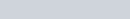Where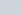is the molar volume of the gas, and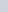is the volume occupied by one mole of the gas.

The second correction term to add is the intermolecular interactions by adding to the pressure. We can now rewrite the equation as: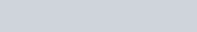This is the Van der Waals equation of state, which can be used for non-ideal gases, like in this example. There are other state functions as well, and the one you should use is dependent on the situation.

## Van der Waals Formula Practice Problems

Problem 1

O2 has the following Van der Waals correction factors: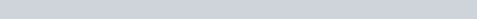You have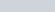of O2 in a rigid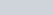container at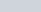. Calculate the pressure.

Problem 2

Gaseous H2O and ethene (C2H4) have the following Van der Waals correction factors: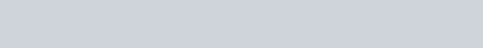At equal temperature, volume, and moles, which gas has the higher pressure?

## Van der Waals Formula Practice Problem Solutions

1: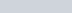2: Ethene